### Generalized Linear Regression

As outlined in the section Generalized Linear Models of Chapter 3: Introduction to Statistical Modeling with SAS/STAT Software, the class of generalized linear model generalizes the linear regression model in two ways:

• by allowing the data to come from a distribution that is a member of the exponential family of distributions

• by introducing a link function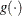that provides a mapping between the linear predictor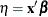and the mean of the data,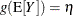. The link function is monotonic, so that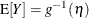and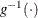is called the inverse link function.

One of the most commonly used generalized linear regression models is the logistic model for binary or binomial data. Suppose that Y denotes a binary outcome variable that takes on the values 1 and 0 with probabilities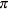and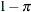, respectively. The probabilityis also referred to as the success probability, supposing that the coding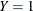corresponds to a success in a Bernoulli experiment. The success probability is also the mean of Y, and one of the aims of logistic regression analysis is to study how regressor variables affect the outcome probabilities or functions thereof, such as odds ratios.

The logistic regression model foris defined by a linear predictorand the logit link function: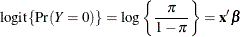The inversely linked linear predictor function in this model is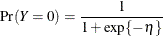An extension of the dichotomous logistic regression model is models for multinomial (polychotomous) data. Two classes of models for multinomial data can be fit with procedures in SAS/STAT software: models for ordinal data that rely on cumulative link functions and models for nominal (unordered) outcomes that rely on generalized logits. The next section briefly discusses SAS/STAT procedures for logistic regression. See Chapter 8: Introduction to Categorical Data Analysis Procedures, for more information about the comparison of the procedures mentioned there with respect to analysis of categorical responses.